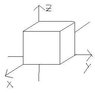# Electric field Q

stunner5000pt
Seems quite strange that i can't solve simple questions like this... but on the other hand applying Gauss Law to cylinders spheres and such isn't that hard for me... ANYWAY
One of the cube's edges is placed at the origin.
A cube with edges 1.4m is oriented as shown in the diagram. Find the flux and the charge inside the cube when the electric field is
a) (3 Nm/c) y j
b) (-4N/C)i + [6N/C + (3Nm/C)y] j

for a) since there are TWO faces in the X only. THe Y and Z componenets are orthogonal to the field thus are zero. $$\Phi = 2 \int E \bullet dA = \frac{q_{enc}}{\epsilon_{0}}$$

$$\Phi = 2 \int 3y [d(y^2)] = 2 \int 6y^2 dy = 4y^3$$
since y = 1.4m flux is 10.976 Nm^2/C
the charge in teh cube as a result is $$10.976=\frac{q_{enc}}{\epsilon_{0}}$$

for b), since there are two faces for the X and Y sides
in the case of the X the field is constnat so $\int E dA = EA$
$$\Phi = 12(1.4)^2 + 2 \int (6+3y)2y dy$$
$$\Phi = 12(1.4)^2 + 2 [6(1.4)^2 + 2(1.4)^3]$$
and plugging that equal to Qenc/epsilon yeilds the induced charge

Am i right? If i am wrong please do tell! Thank you so much!

#### Attachments

•charge.JPG
4.2 KB · Views: 334
Last edited:

Homework Helper
The way I read #1(a), the Field is parallel
to the y-axis (you use the j_vec),
which would pierce the Y-faces
except that one of these is at y = 0.

E_vec must *pierce* the surface area,
so you want dA = dxdz
..(dy is parallel dy, so can't make an area)
take E dot (dx cross dz)

stunner5000pt
im not quite sure about what you just said...
but for the E field in 1a isn't the EdA cos0?
And the other side EA cos 180??
could you explain more in depth?

Homework Helper
yes, the dot product gives EdAcos0,
at the y = 1.4 [m] face ...
where A = Dx * Dz (not the Dy^2 you were using).

For the y = 0 face , well y=0 so E=0.

The E-field at each face is different, so you can't just multiply the integral by 2.
And the dA is nothing at all like d(y^2) .

You make the same 2 mistakes in part (b),
where you conclude that the uniform Ex-component
indicates the presence of a lot of charge in the box.
uniform E means zero charge in the region;
charges cause E-field lines to diverge.

stunner5000pt
ok so for hte first one then
$$\Phi = \int E \bullet dA = \int E \bullet dx dz$$
the area vector is the cross product of these two vectors
Can be a scalar?? So A = 1.4^2
or should it be in vector form?

stunner5000pt
so dx cross dz will give me dy
so dx = (1.4,0,0)
and dz = (0,0,1.4)
is this correct
because the cross product of these two would yield (0,1.96,0) is this correct?

anbd then i dot this with the electric field? SO the integral sign goes away? Since ifound a numerical value for dy?
so the flux is 3(1.96)(1.4)?? AM i on the right track?